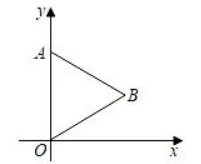$\text{A.}$ $(2 \sqrt{3}, 2)$ $\text{B.}$ $(2 \sqrt{3},-2)$ $\text{C.}$ $(-2 \sqrt{3},-2)$ $\text{D.}$ $(0,-4)$